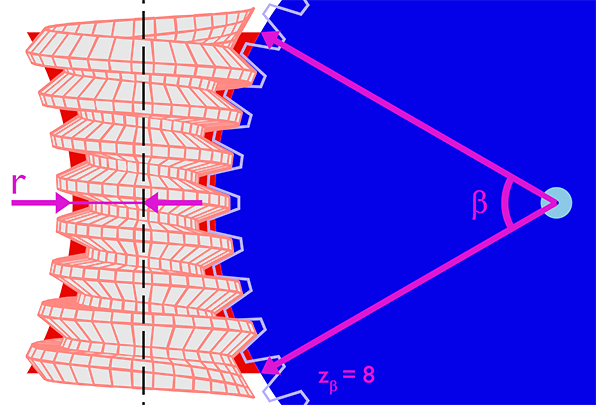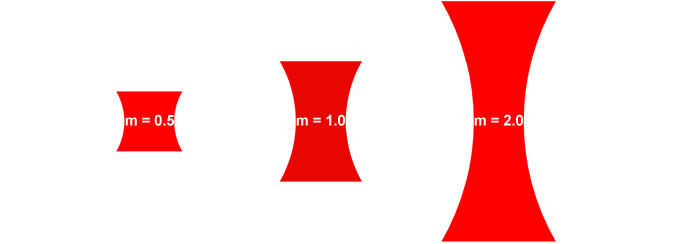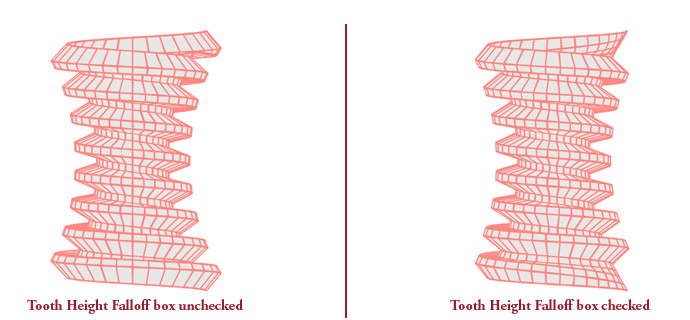Home  •  Tutorials  •  Calculators  •  3D Models  •  About  •  Contact

Globoid Worm Shaft Calculator

The following online calculator computes the parametric equations of a globoid (throated) worm shaft based on its basic dimensions, shape and desired number of teeth in the matching gear wheel.A globoid worm shaft is based on an hourglass shape cut out of a torus (shown in red.) Its dimensions are defined by the radius of its narrowest section (r), angular size of its arc (β) and the diameter of the corresponding gear wheel. The latter is calculated as the total number of teeth multiplied by the user-specified module (m). The total number of teeth is in turn calculated based on the user-specified number of teeth that fall within the hourglass's shape (8 in this example). If β is 60°, the total number of teeth in the wheel is 360° / β * 8 = 48. If the module is 1mm, the wheel diameter is 48mm. A module value less than 1 makes the worm shaft squatter and the wheel smaller, while a value greater than 1 makes the shaft taller and the wheel larger, as shown on the image below:The effect of the Tooth Height Falloff checkbox is demonstrated on the image below. If the box is unchecked the worm's tooth height remains unchanged throughout the entire length of the spiral. This is useful when the worm's surface is used to model the tooth profile for the matching gear wheel. If the box is checked, the tooth height falls off gradually at the top and bottom of the spiral to become flush with the hourglass surface. This option should be used for the final product.Basic Dimensions
ItemSymbolValue
Modulem
Arc Angleβ
Gear Teeth within the Arczβ